Next: References Up: FORMALIZATION OF TWO PUZZLES Previous: AXIOMATIZATION OF THE WISE

# AXIOMATIZATION OF MR. S AND MR. P

These axioms involve the same ideas as the wise man axiomatization. They are a debugged version of the axioms by Ma Xiwen of Peking University, which, in turn, were a variant of my earlier axiomatization.

This formalization separates the knowledge part of the problem from the arithmetic part in a neat way. Ma Xiwen verified, using FOL, that his axioms imply a certain purely arithmetic condition on the pair of numbers. It can be then shown that the only pair satisfying that condition is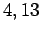.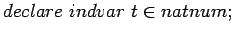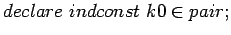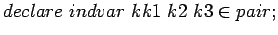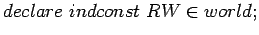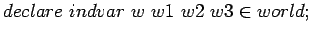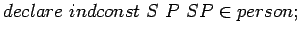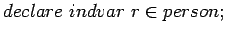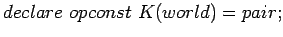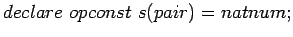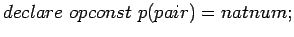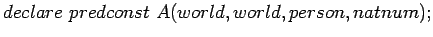The predicates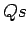,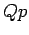,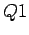,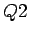,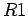,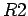and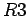are represent arithmetic conditions on the pair of numbers that are used to express the arithmetic conditions on the pair that replace the knowledge conditions given in the problem statement.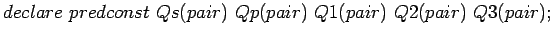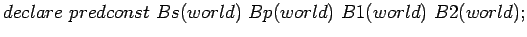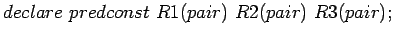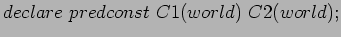The first two axioms state that the accessibility relation is reflexive and transitive. They assert that what is known is true and one knows that one knows what one knows. Got that?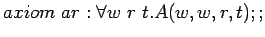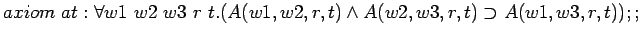This axiom says that the joint person knows what Mr. S and Mr. P both know. At first sight it seems too weak, but transitivity tells us that what they jointly know, they jointly know they jointly know.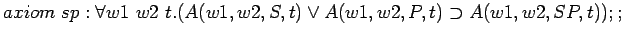This next axiom is just a definition for the purposes of abbreviation. RW is the real world, so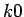is just the real pair.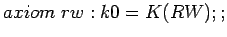In the initial situation they jointly know that Mr. S knows the sum and Mr. P the product. They also jointly know that this is all that Mr. S and Mr. P know about the numbers. This is asserted by saying that given any pair of numbers with the right sum, there is a world possible for Mr. S in which this is the pair of numbers.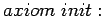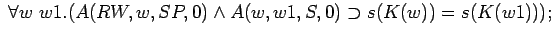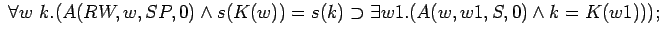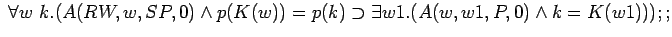These axioms on the pair will be used to translate knowledge assertions. For example,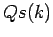asserts that there is another pair that has the same sum as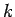and is used in the assertion that Mr. S, knowing the sum, does not know the pair, i.e. does not know the numbers. As we proceed through the dialog the arithmetic conditions become more complex.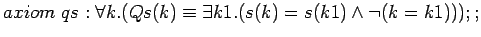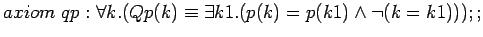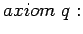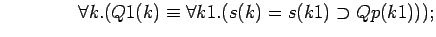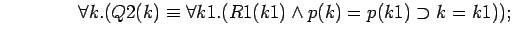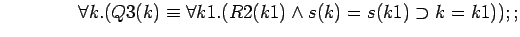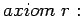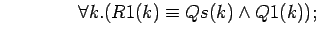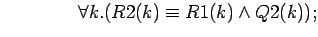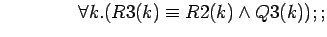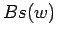asserts that in the possible world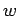, Mr. S doesn't know the numbers.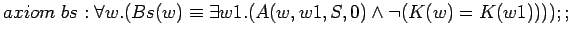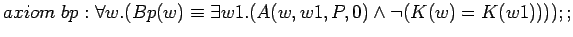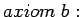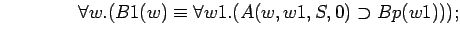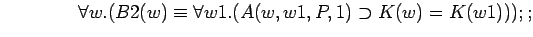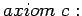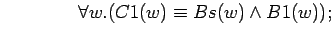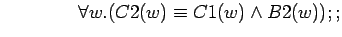The previous axioms were just definitions. Now we have the information coming from the dialog. SKNPK stands for S Knows that P does Not Know'' and the axiom asserts this about the real world at time.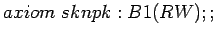NSK stands for S doesn't know'', and the axiom asserts this about the real world.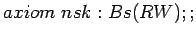PK stands for P knows the numbers'', and the axiom asserts this about the real world at time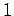.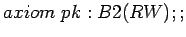SK tells us that at time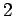, Mr. S knows the numbers.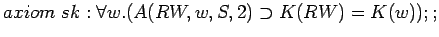The last two axioms are the learning axioms. LS tells us that everyone learns by timethat Mr. S knew at timethat Mr. P didn't know the numbers, and he didn't know them either. LP tells us that everyone learns by time 2 that Mr. P knew the numbers by time.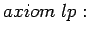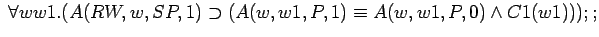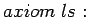Ma Xiwen's proof was carried out using a sequence of lemmas. The first lemma shows the essential equivalence of a condition on possible worlds with a condition on pairs.

Lemma 1.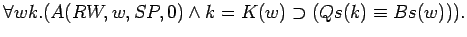Lemma 2.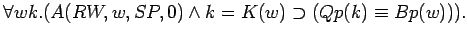Lemma 3.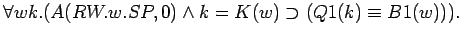Lemma 4.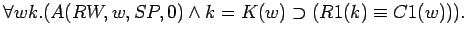Lemma 5.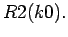Lemma 6.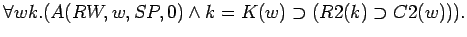Lemma 7.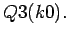Main Theorem.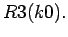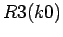is a purely arithmetic condition on the pair of numbers. From an axiom asserting thatis a pair of numbers in the intervaland Peano's axioms for arithmetic, the numbers can be proved to be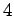and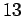. Alternatively,can be translated into a computer program for computing the numbers. Most people who solve the problem write such a program.Next: References Up: FORMALIZATION OF TWO PUZZLES Previous: AXIOMATIZATION OF THE WISE
John McCarthy
2007-11-19